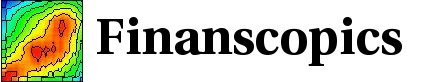Base information Indexes

# Probability density function for "Constant volatility with Student innovations"

## Pdf for the return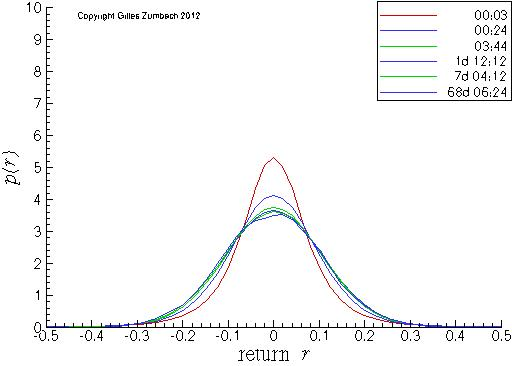Probability density for the return, in linear-linear scales.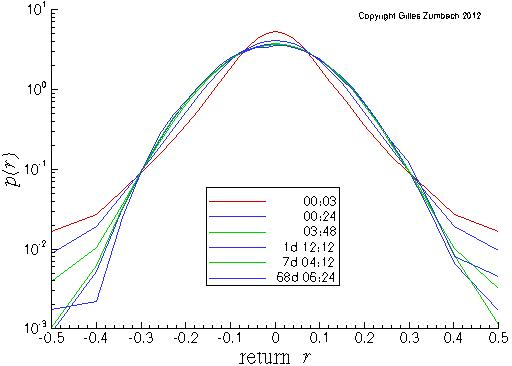Probability density for the return, in linear-logarithmic scales.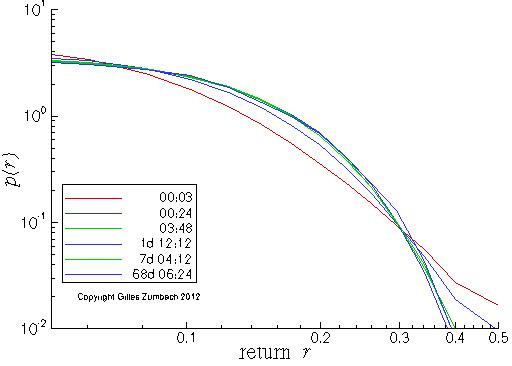Probability density for the return, in log-log scales, for the positive returns.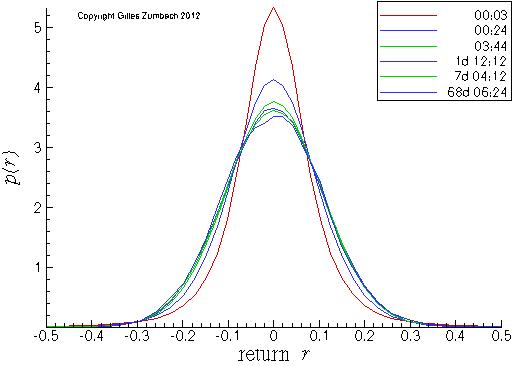Probability density for the return, in linear-linear scales.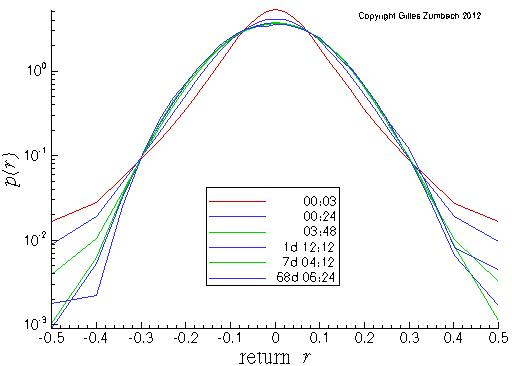Probability density for the return, in linear-logarithmic scales.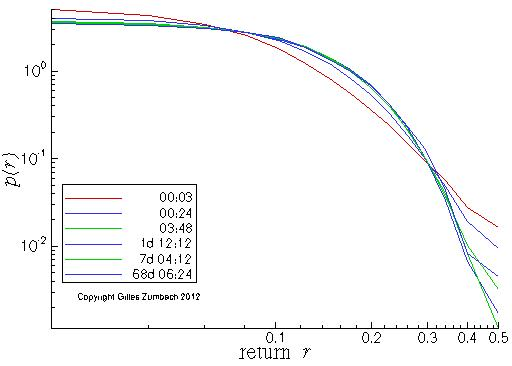Probability density for the return, in log-log scales, for the positive returns.

## Pdf for the volatility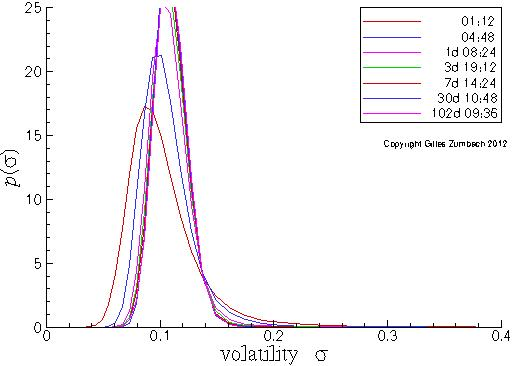Probability density for the volatility, in lin-lin scales.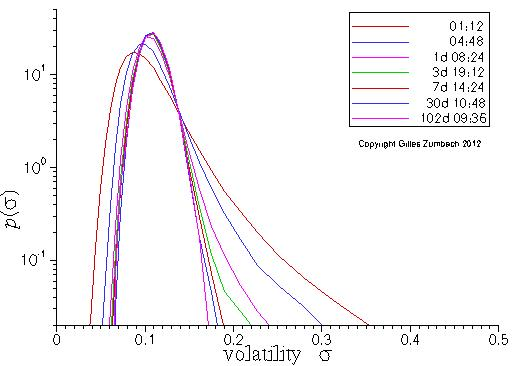Probability density for the volatility, in lin-log scales.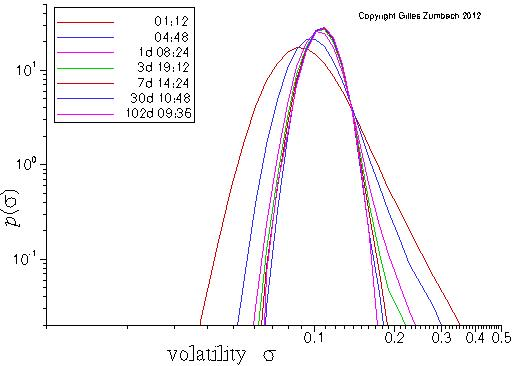Probability density for the volatility, in log-log scales.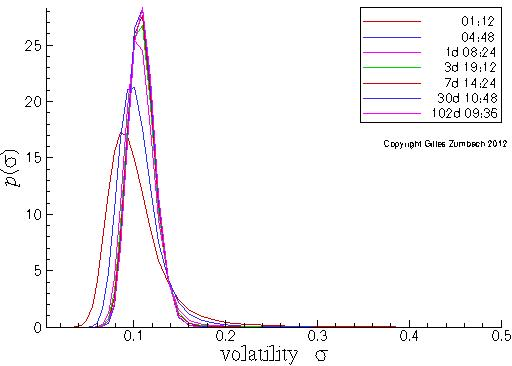Probability density for the volatility, in lin-lin scales.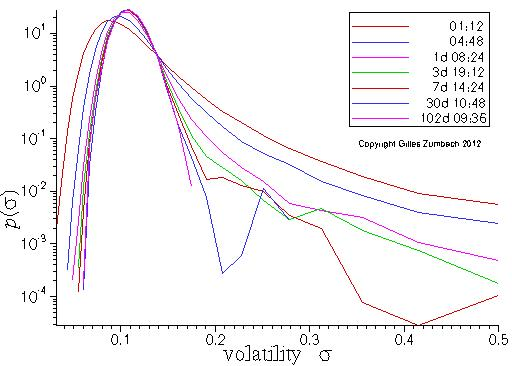Probability density for the volatility, in lin-log scales.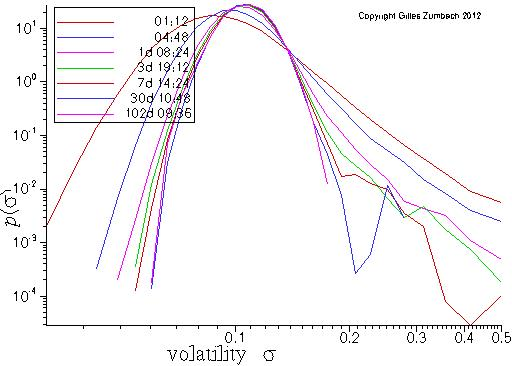Probability density for the volatility, in log-log scales.

## Pdf for the volatility increment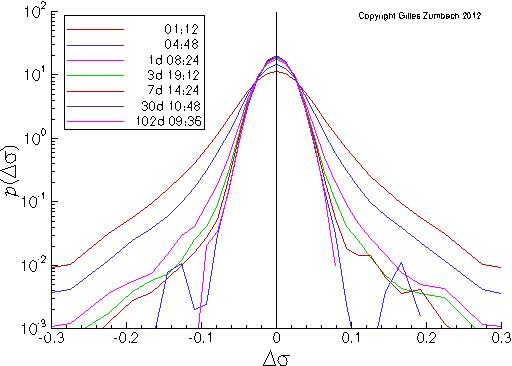Probability density for the volatility increment, in lin-log scales.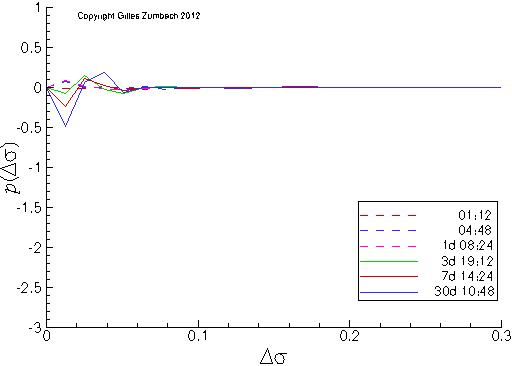Probability density asymmetry for the volatility increment.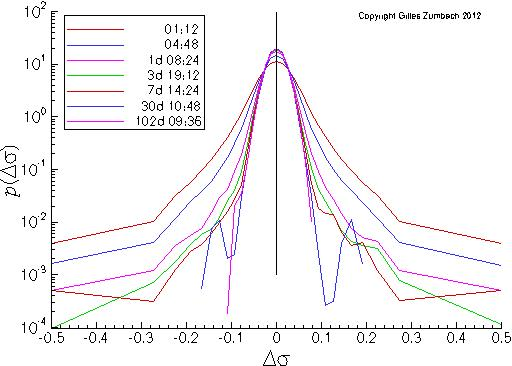Probability density for the volatility increment, in lin-log scales.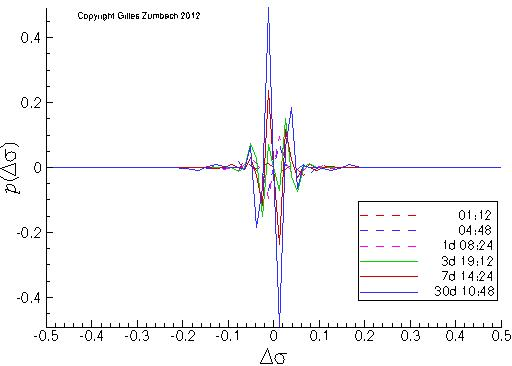Probability density asymmetry for the volatility increment.